# # d3-geo

``````function mercator(x, y) {
return [x, Math.log(Math.tan(Math.PI / 4 + y / 2))];
}
``````

D3的方法提供了很好的表现力: 你可以为你的数据选择正确的投影和样貌。D3 支持许多常见的投影以及 特殊的地理投影 (opens new window). 更多信息可以参考 工具制作指南 (opens new window).

D3 使用 GeoJSON (opens new window) 来表现地理特征. (也可以参考TopoJSON (opens new window), 一种更加紧密的对 GeoJSON 的编码). 可以使用 shp2geo (opens new window) 将 shape 文件转为 GeoJSON, 这是 shapefile package (opens new window) 的一部分. 有关 d3-geo 和相关工具的介绍，请参阅 Command-Line Cartography (opens new window).

## # Installing

``````<script src="https://d3js.org/d3-array.v1.min.js"></script>
<script src="https://d3js.org/d3-geo.v1.min.js"></script>
<script>

var projection = d3.geoNaturalEarth1(),
path = d3.geoPath(projection);

</script>
``````

## # Paths

# d3.geoPath([projection[, context]]) <> (opens new window)

# path(object[, arguments…]) <> (opens new window)

• Point - 单个点.
• MultiPoint - 一组点集.
• LineString - 一组点集表示的连续的线.
• MultiLineString - 组点集数组表示的多条线.
• Polygon - 点集数组表示的多边形(可能有镂空).
• MultiPolygon - 表示多个多边形的点集.
• GeometryCollection - 一组几何对象.
• Feature - 包含上述几何对象之一的特征.
• FeatureCollection - 一组集合特征.

``````svg.append("path")
.datum({type: "FeatureCollection", features: features})
.attr("d", d3.geoPath());
``````

``````svg.selectAll("path")
.data(features)
.enter().append("path")
.attr("d", d3.geoPath());
``````

# path.area(object) <> (opens new window)

# path.bounds(object) <> (opens new window)

# path.centroid(object) <> (opens new window)

# path.measure(object) <> (opens new window)

# path.projection([projection]) <> (opens new window)

# path.context([context]) <> (opens new window)

• context.beginPath()
• context.moveTo(x, y)
• context.lineTo(x, y)
• context.arc(x, y, radius, startAngle, endAngle)
• context.closePath()

## # Projections

# projection(point) <> (opens new window)

# projection.invert(point) <> (opens new window)

# projection.stream(stream) <> (opens new window)

# projection.preclip([preclip])

# projection.postclip([postclip])

# projection.clipAngle([angle]) <> (opens new window)

# projection.clipExtent([extent]) <> (opens new window)

# projection.scale([scale]) <> (opens new window)

# projection.translate([translate]) <> (opens new window)

# projection.center([center]) <> (opens new window)

# projection.angle([angle]) <> (opens new window)

# projection.rotate([angles]) <> (opens new window)

# projection.precision([precision]) <> (opens new window)

# projection.fitExtent(extent, object) <> (opens new window)

``````var projection = d3.geoTransverseMercator()
.rotate([74 + 30 / 60, -38 - 50 / 60])
.fitExtent([[20, 20], [940, 480]], nj);
``````

# projection.fitSize(size, object) <> (opens new window)

projection.fitExtent 的便捷用法, 其中左上角坐标为 [0, 0]. 下面两个用法是等效的:

``````projection.fitExtent([[0, 0], [width, height]], object);
projection.fitSize([width, height], object);
``````

# projection.fitWidth(width, object) <> (opens new window)

projection.fitSize 的便捷用法, 其中高度是根据对象的宽高比和宽度自动计算的.

# projection.fitHeight(height, object) <> (opens new window)

projection.fitSize 的便捷用法, 其中宽度是根据对象的宽高比和高度自动计算的.

## # Azimuthal Projections

# d3.geoAzimuthalEqualArea() <> (opens new window)
# d3.geoAzimuthalEqualAreaRaw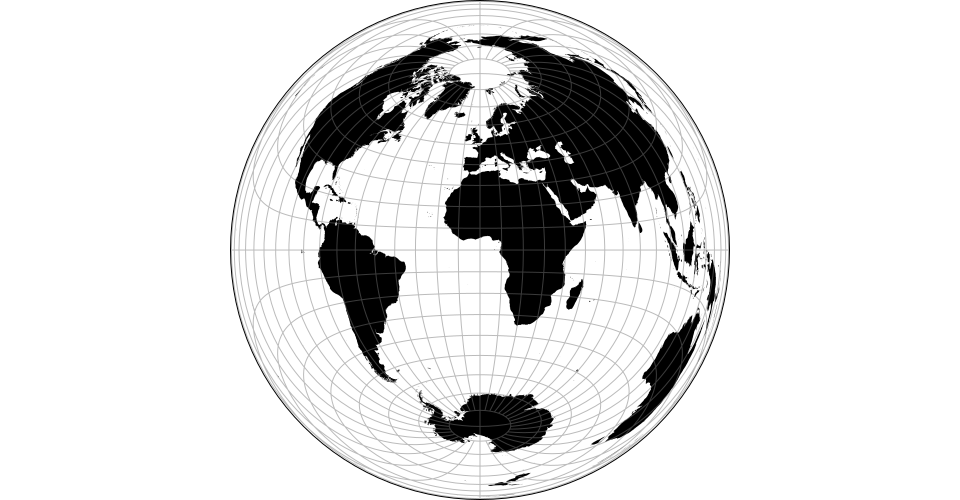(opens new window)

# d3.geoAzimuthalEquidistant() <> (opens new window)
# d3.geoAzimuthalEquidistantRaw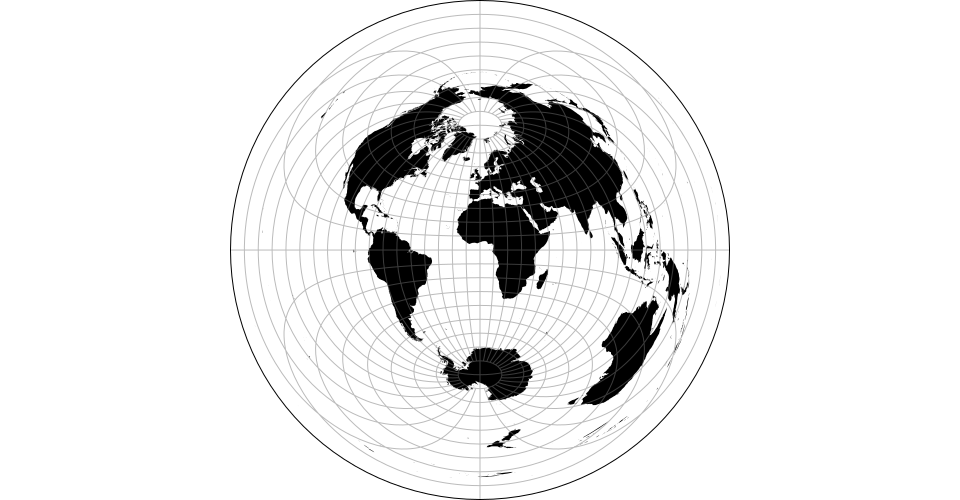(opens new window)

# d3.geoGnomonic() <> (opens new window)
# d3.geoGnomonicRaw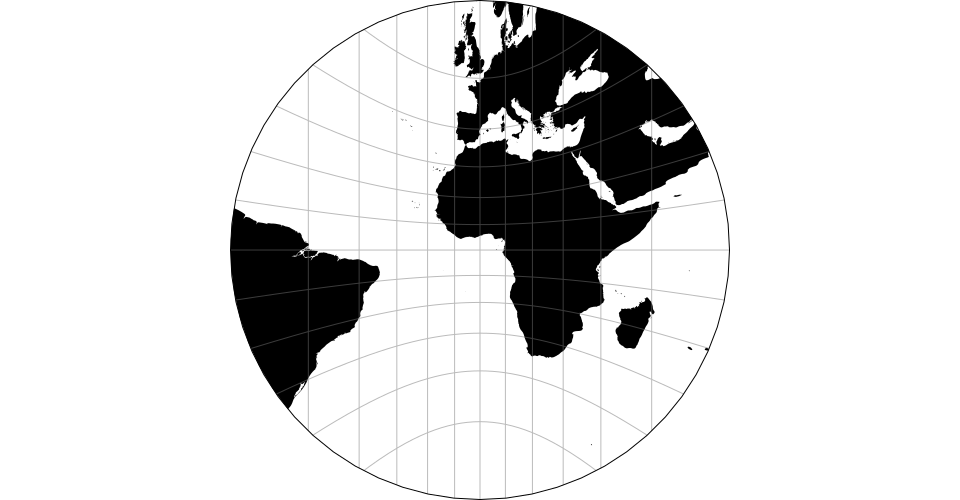(opens new window)

# d3.geoOrthographic() <> (opens new window)
# d3.geoOrthographicRaw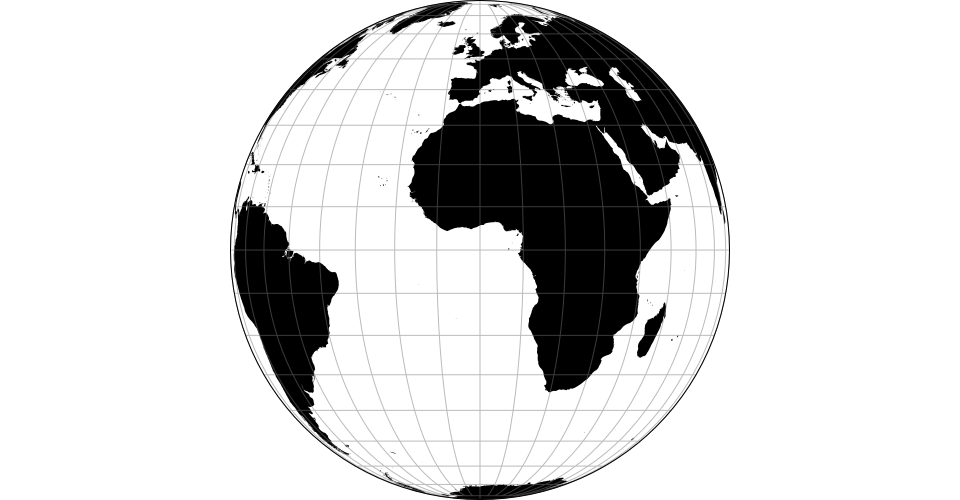(opens new window)

# d3.geoStereographic() <> (opens new window)
# d3.geoStereographicRaw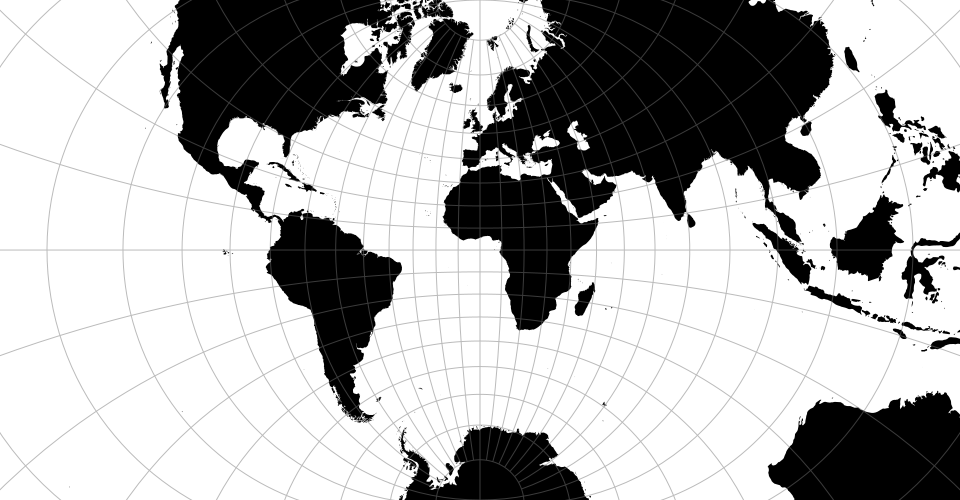(opens new window)

## # Composite Projections

# d3.geoAlbersUsa() <> (opens new window)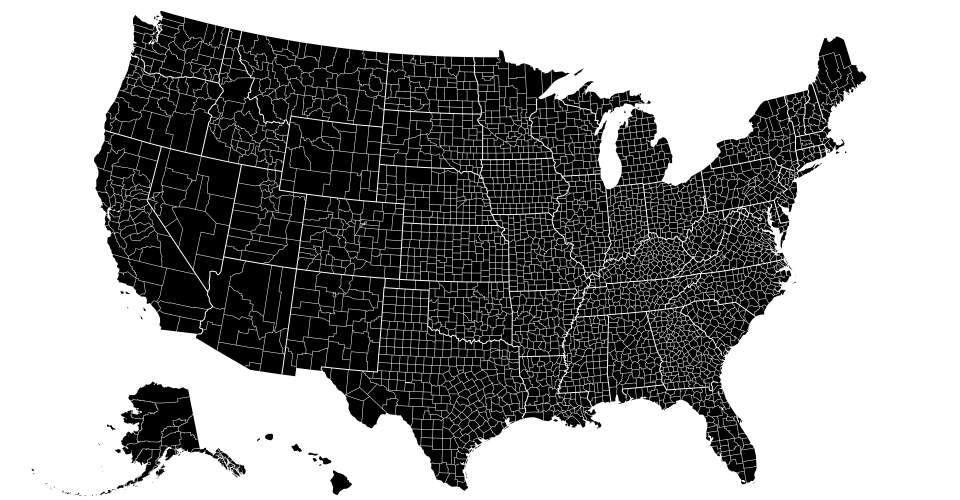(opens new window)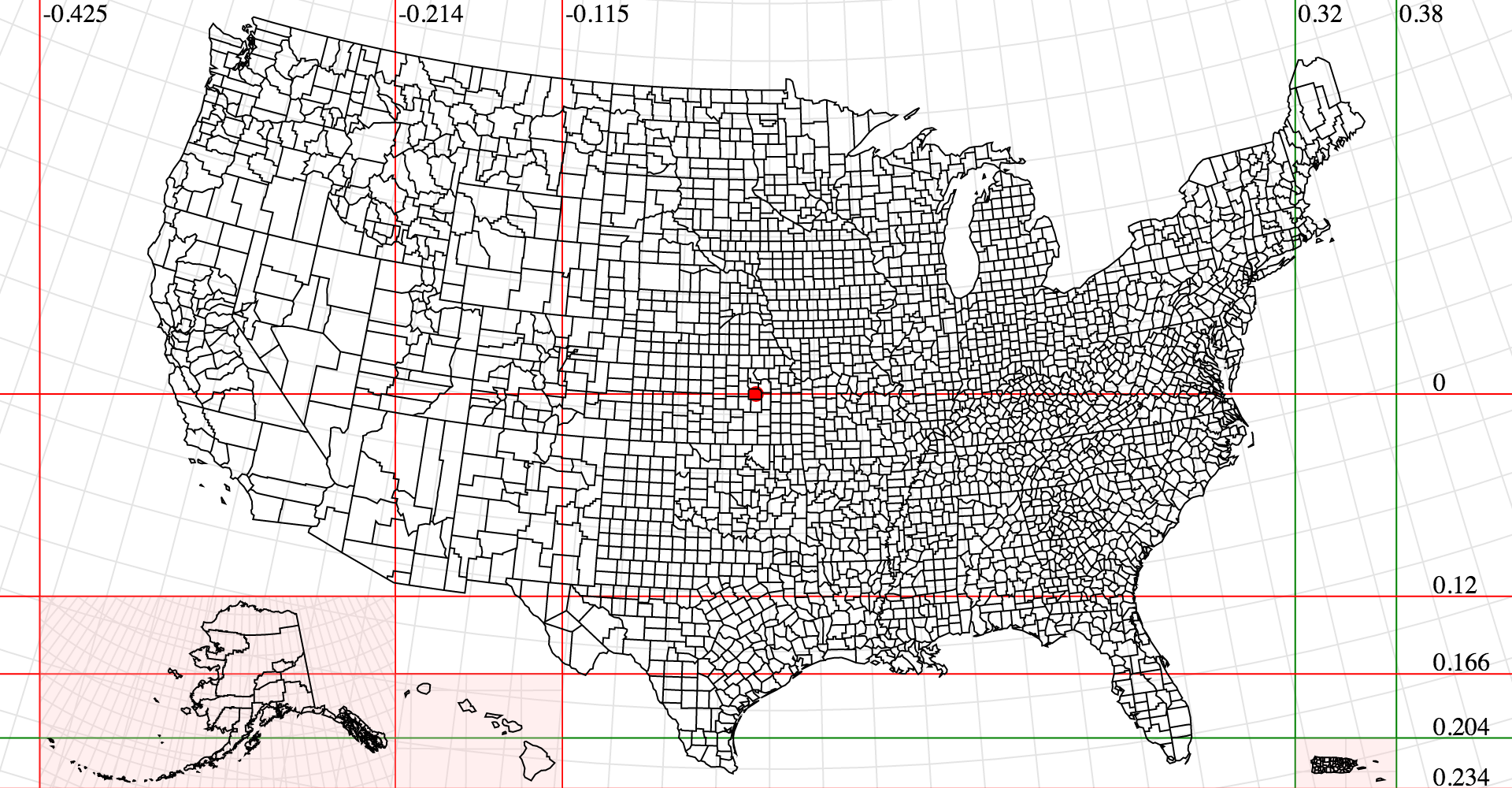(opens new window)

See d3-composite-projections (opens new window) for more examples.

## # Conic Projections

# conic.parallels([parallels]) <> (opens new window)

# d3.geoAlbers() <> (opens new window)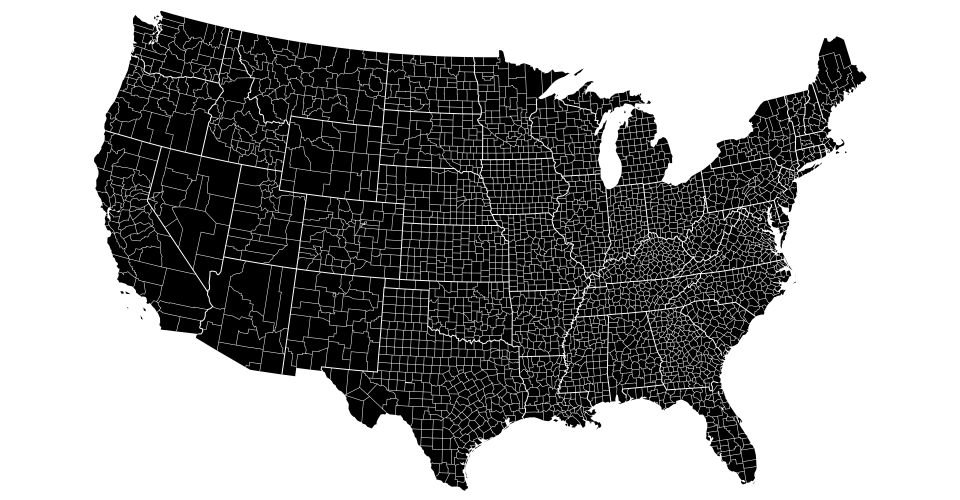(opens new window)

`Albers` 的等面积圆锥投影. 这是以美国为中心的 d3.geoConicEqualArea.

# d3.geoConicConformal() <> (opens new window)
# d3.geoConicConformalRaw(phi0, phi1) <> (opens new window)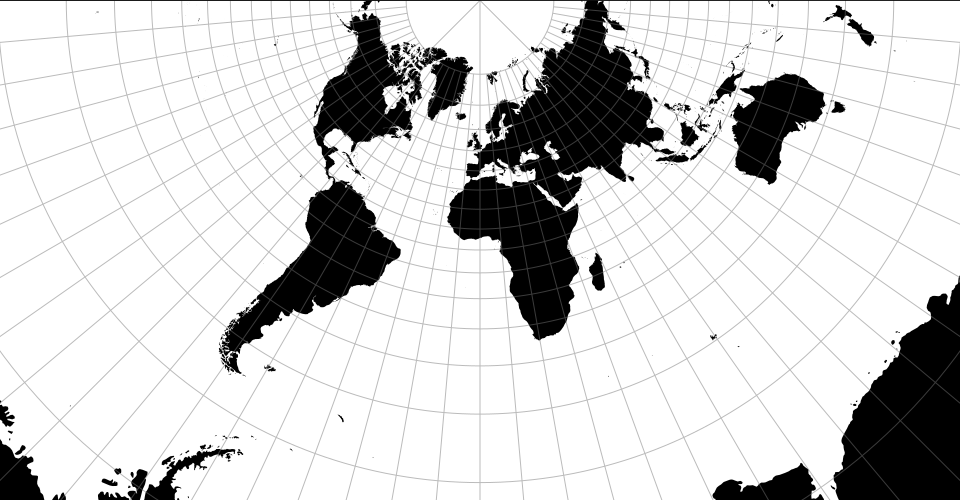(opens new window)

# d3.geoConicEqualArea() <> (opens new window)
# d3.geoConicEqualAreaRaw(phi0, phi1) <> (opens new window)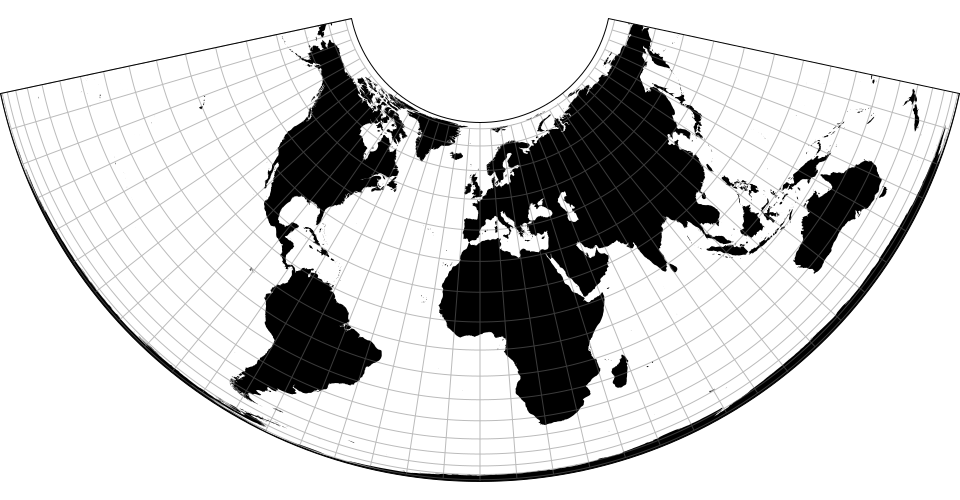(opens new window)

`Albers` 等面积圆锥投影. 参考 conic.parallels.

# d3.geoConicEquidistant() <> (opens new window)
# d3.geoConicEquidistantRaw(phi0, phi1) <> (opens new window)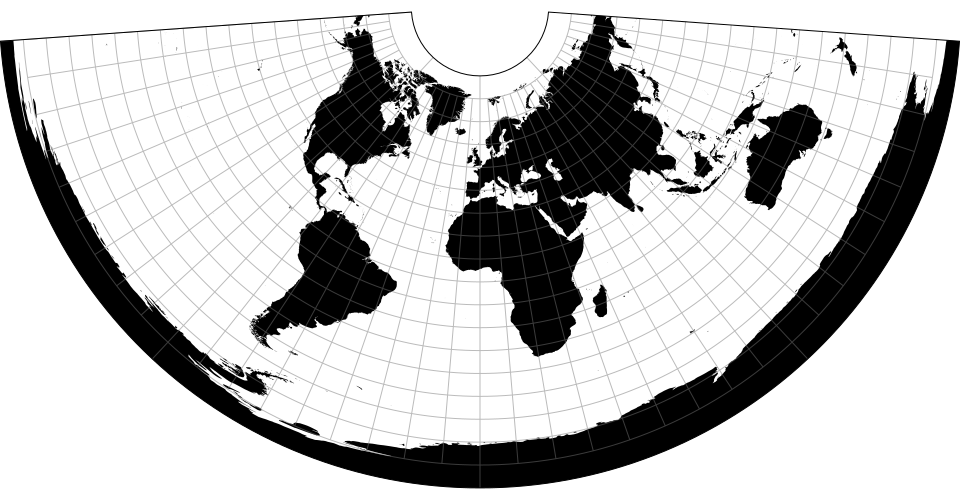(opens new window)

## # Cylindrical Projections

# d3.geoEquirectangular() <> (opens new window)
# d3.geoEquirectangularRaw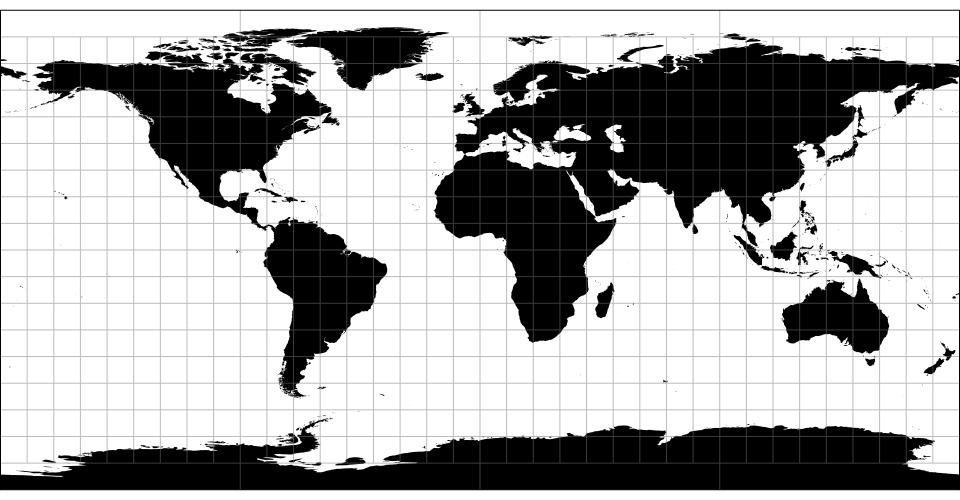(opens new window)

# d3.geoMercator() <> (opens new window)
# d3.geoMercatorRaw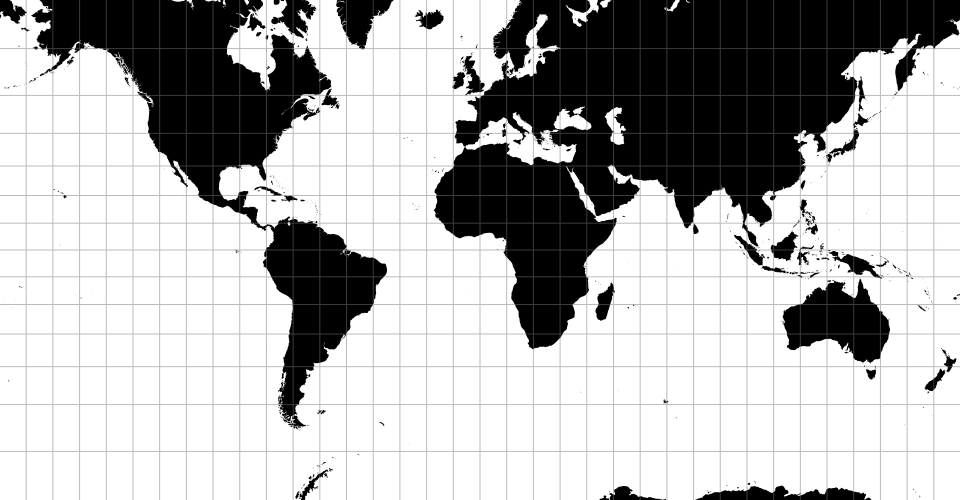(opens new window)

# d3.geoTransverseMercator() <> (opens new window)
# d3.geoTransverseMercatorRaw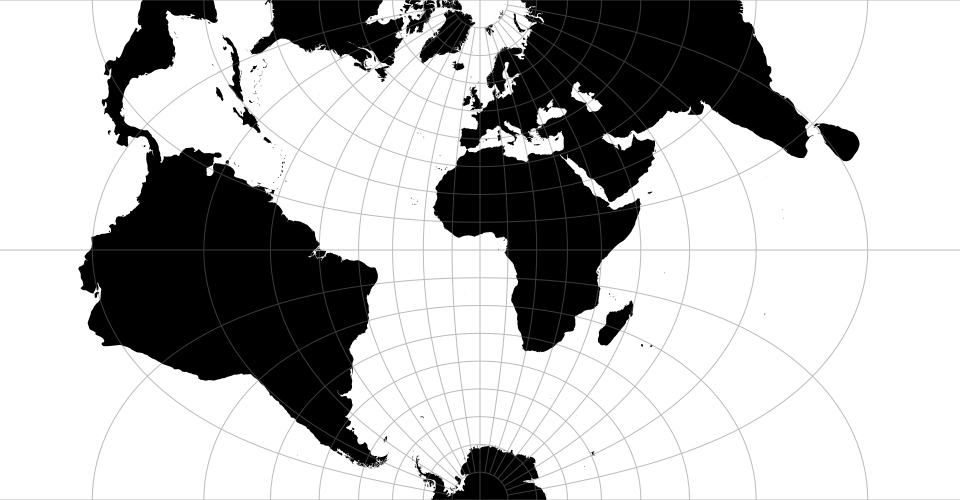(opens new window)

# d3.geoNaturalEarth1() <> (opens new window)
# d3.geoNaturalEarth1Raw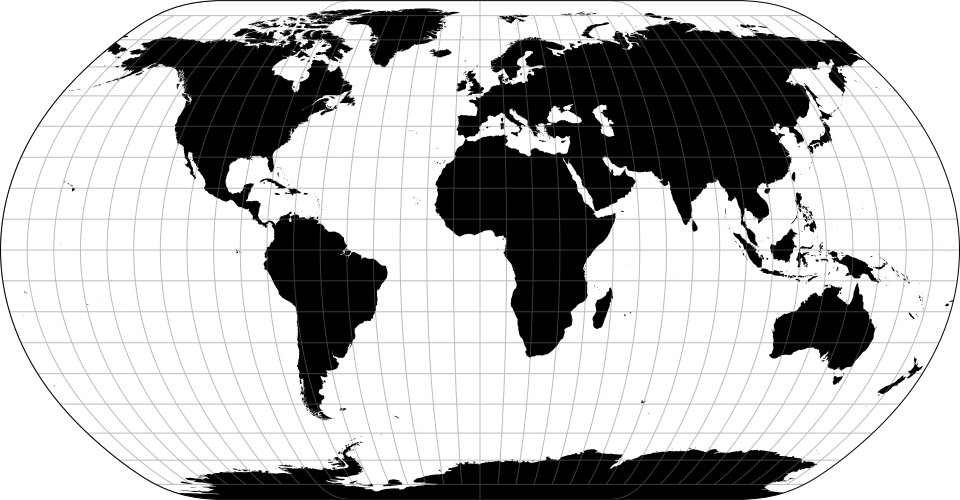(opens new window)

Natural Earth projection(自然地球投影) (opens new window) 是由 `Tom Patterson` 设计的伪圆柱投影. 它既不是等角也不是等角的, 但是看起来很吸引人, 像是一个缩小的世界.

## # Raw Projections

# project(lambda, phi)

# project.invert(x, y)

project 的逆计算.

# d3.geoProjection(project) <> (opens new window)

``````var mercator = d3.geoProjection(function(x, y) {
return [x, Math.log(Math.tan(Math.PI / 4 + y / 2))];
});
``````

# d3.geoProjectionMutator(factory) <> (opens new window)

``````// y0 and y1 represent two parallels
function conicFactory(phi0, phi1) {
return function conicRaw(lambda, phi) {
return […, …];
};
}
``````

``````function conicCustom() {
var phi0 = 29.5,
phi1 = 45.5,
mutate = d3.geoProjectionMutator(conicFactory),
projection = mutate(phi0, phi1);

projection.parallels = function(_) {
return arguments.length ? mutate(phi0 = +_, phi1 = +_) : [phi0, phi1];
};

return projection;
}
``````

## # Spherical Math

# d3.geoArea(object) <> (opens new window)

# d3.geoBounds(object) <> (opens new window)

# d3.geoCentroid(object) <> (opens new window)

# d3.geoDistance(a, b) <> (opens new window)

# d3.geoLength(object) <> (opens new window)

# d3.geoInterpolate(a, b) <> (opens new window)

# d3.geoContains(object, point) <> (opens new window)

# d3.geoRotation(angles) <> (opens new window)

# rotation(point) <> (opens new window)

# rotation.invert(point) <> (opens new window)

## # Spherical Shapes

# d3.geoCircle() <> (opens new window)

# circle(arguments…) <> (opens new window)

# circle.center([center]) <> (opens new window)

``````function center() {
return [0, 0];
}
``````

``````function radius() {
return 90;
}
``````

# circle.precision([angle]) <> (opens new window)

``````function precision() {
return 6;
}
``````

# d3.geoGraticule() <> (opens new window)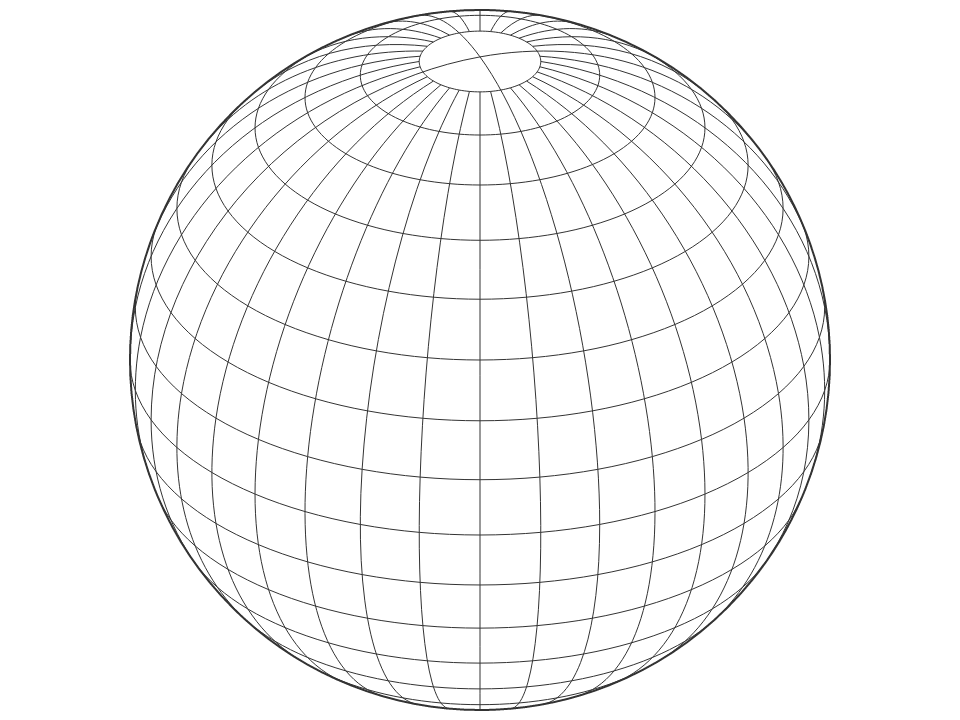# graticule() <> (opens new window)

# graticule.lines() <> (opens new window)

# graticule.outline() <> (opens new window)

# graticule.extent([extent]) <> (opens new window)

# graticule.extentMajor([extent]) <> (opens new window)

# graticule.extentMinor([extent]) <> (opens new window)

# graticule.step([step]) <> (opens new window)

# graticule.stepMajor([step]) <> (opens new window)

# graticule.stepMinor([step]) <> (opens new window)

# graticule.precision([angle]) <> (opens new window)

# d3.geoGraticule10() <> (opens new window)

``````function geoGraticule10() {
return d3.geoGraticule()();
}
``````

## # Streams

`D3` 使用一系列函数调用进行几何转换, 过渡过程中的中间值不被保存以最小化开销. 流必须实现多个方法来接收输入几何图形. 流本质上是有状态的; point 的定义取决于该点是否在 line 内, 同样地线与环的区别是由 polygon 决定的. 尽管被命名为 “流” 但是这些方法是被同步调用的.

# d3.geoStream(object, stream) <> (opens new window)

# stream.point(x, y[, z])

# stream.lineStart()

# stream.lineEnd()

``````{
"type": "Polygon",
"coordinates": [
[[0, 0], [0, 1], [1, 1], [1, 0], [0, 0]]
]
}
``````

``````stream.polygonStart();
stream.lineStart();
stream.point(0, 0);
stream.point(0, 1);
stream.point(1, 1);
stream.point(1, 0);  // 结束，不会调用 stream.point(0, 0)
stream.lineEnd();
stream.polygonEnd();
``````

# stream.polygonStart()

# stream.polygonEnd()

# stream.sphere()

## # Transforms

# d3.geoTransform(methods) <> (opens new window)

``````var reflectY = d3.geoTransform({
point: function(x, y) {
this.stream.point(x, -y);
}
});
``````

``````function matrix(a, b, c, d, tx, ty) {
return d3.geoTransform({
point: function(x, y) {
this.stream.point(a * x + b * y + tx, c * x + d * y + ty);
}
});
}
``````

# d3.geoIdentity() <> (opens new window)

# identity.reflectX([reflect])

# identity.reflectY([reflect])

## # Clipping

# preclip(stream)

# postclip(stream)

# d3.geoClipAntimeridian

# d3.geoClipCircle(angle)

# d3.geoClipRectangle(x0, y0, x1, y1)